Tapping into mathematics

Start this free course now. Just create an account and sign in. Enrol and complete the course for a free statement of participation or digital badge if available.

Free course

# 5.5 Comparing price rises

While reading a newspaper article, I noticed some examples of how prices changed in the 10 years from 1984 to 1994. The table below shows the typical prices that you would have expected to pay in 1984 and in 1994 for a pint of milk and a Ford Fiesta motor car.

Price in 1984Price in 1994
Pint of milk21 pence36 pence
New Ford Fiesta£4704£7990

I was interested to see whether the price of milk had increased more than the price of Ford Fiestas. One approach might be to subtract the 1984 price from the 1994 price to give the price increase. This reveals that milk rose in price by 15 pence (36 pence – 21 pence) while Ford Fiestas rose in price by £3286 (£7990 − £4704). However, this is not a very helpful result, as I am not comparing like with like. A more useful approach is to calculate the percentage price increases of each item over the ten-year period. Here are two possible ways of calculating the percentage price increase of a pint of milk.

Method 1

The increase in price of a pint of milk was 36p − 21p = 15p. Now this increase can be expressed as a percentage by writing it as a fraction of the original price and multiplying it by 100: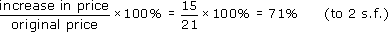Method 2

You can work out the new price as a percentage of the original price by expressing the new price as a fraction of the old price and multiplying by 100: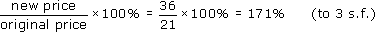Since the original price is 100%, the percentage price increase is approximately 171% − 100% = 71%.

So whichever way you calculate it, milk prices went up by 71% over the 10-year period.

## Exercise 7: Pinta or fiesta?

Make sure that you understand how the above calculations were done.

• (a) Use you calculator to work out the percentage price increase of a Ford Fiesta.

• (b) Which of the two items, milk or Ford Fiesta, has shown the greatest percentage price rise?

• (a) For the car, increase in price £7990 − £4704 = £3286.

Method 1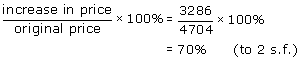Method 2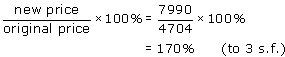So the percentage price increase is 170% − 100% = 70%

The above calculations appeared on the calculator (set to two decimal places) as shown here: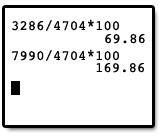• (b) The percentage increase of the pint of milk was very slightly more than that of the car.

MU120_4MCAL